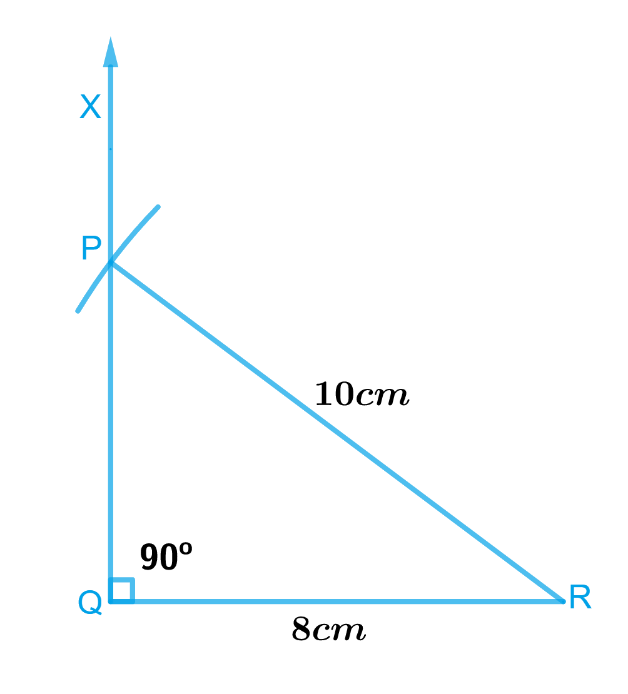# Ex.10.5 Q1 Practical Geometry Solution- NCERT Maths Class 7

Go back to  'Ex.10.5'

## Question

Construct the right angled $$ΔPQR$$, where $$m∠Q = 90^\circ$$, $$QR = 8 \rm{cm}$$ and $$PR = 10\, \rm{cm}.$$

Video Solution
Practical Geometry
Ex 10.5 | Question 1

## Text Solution

What is known?

Length of two side of a right angled $$ΔPQR$$.

To construct:

A right angled $$ΔPQR$$, where $$∠Q = 90^\circ$$, $$QR = 8 \rm{cm}$$ and $$PR = 10\, \rm{cm}.$$

Reasoning:

To construct a right-angled $$ΔPQR$$, where $$∠Q = 90^\circ$$, $$QR = 8\,\rm{cm}$$ and $$PR = 10 \,\rm{cm}$$, draw a rough sketch and mark the measures. Remember to mark the right angle and follow the steps given below.

Steps:Steps of construction :

1. Draw $$QR$$ of length $$8\, \rm{cm}.$$
2. At $$Q$$, draw $$QX\,\text{perpendicular}\,QR.$$
3. With $$R$$ as centre, draw an arc of radius $$10\,\rm{ cm}$$ which should intersect $$QX$$ at point $$P$$.
4. Join $$P$$ and $$R$$.

$$ΔPQR$$ is the required triangle.

Learn from the best math teachers and top your exams

• Live one on one classroom and doubt clearing
• Practice worksheets in and after class for conceptual clarity
• Personalized curriculum to keep up with school